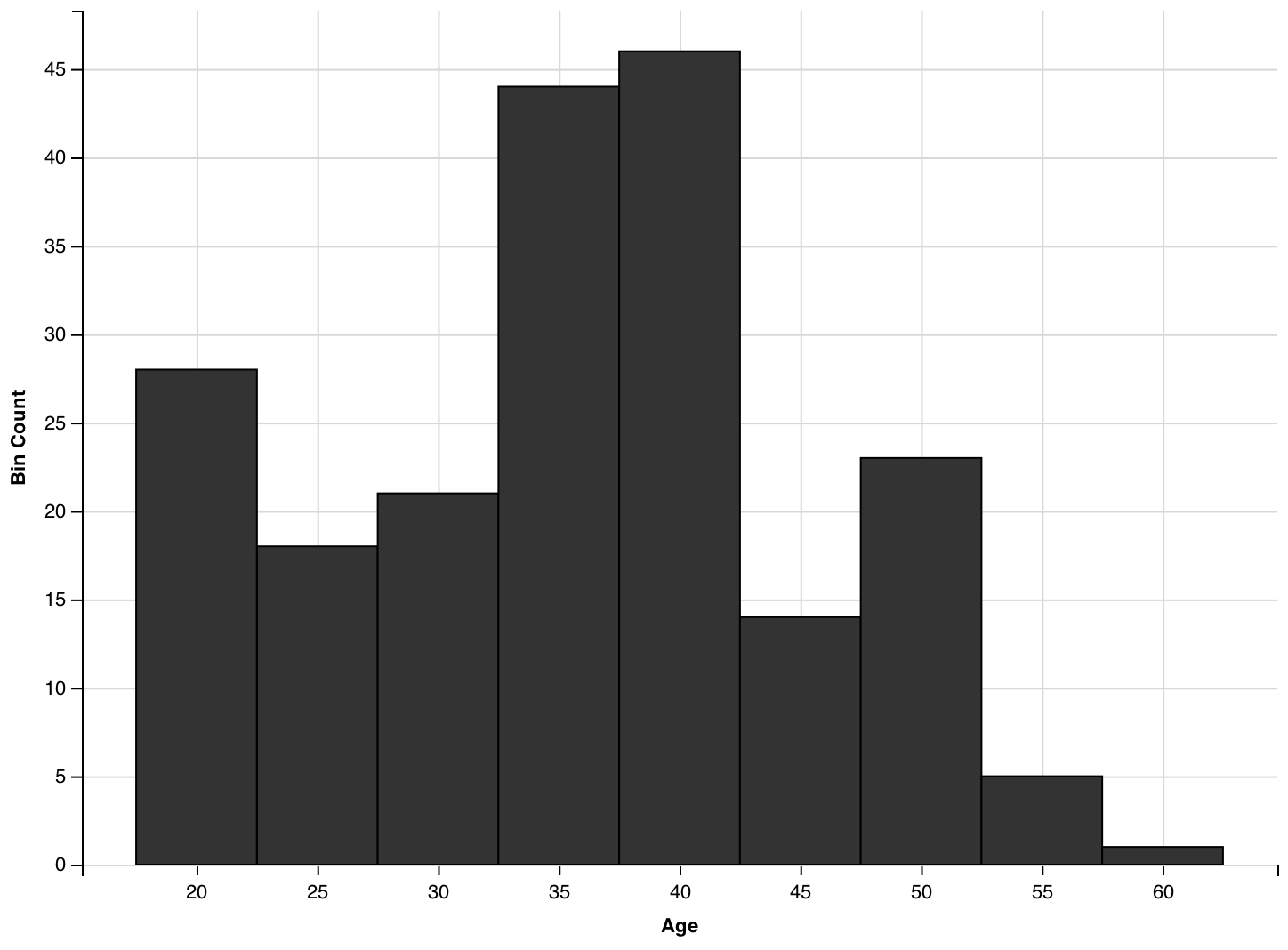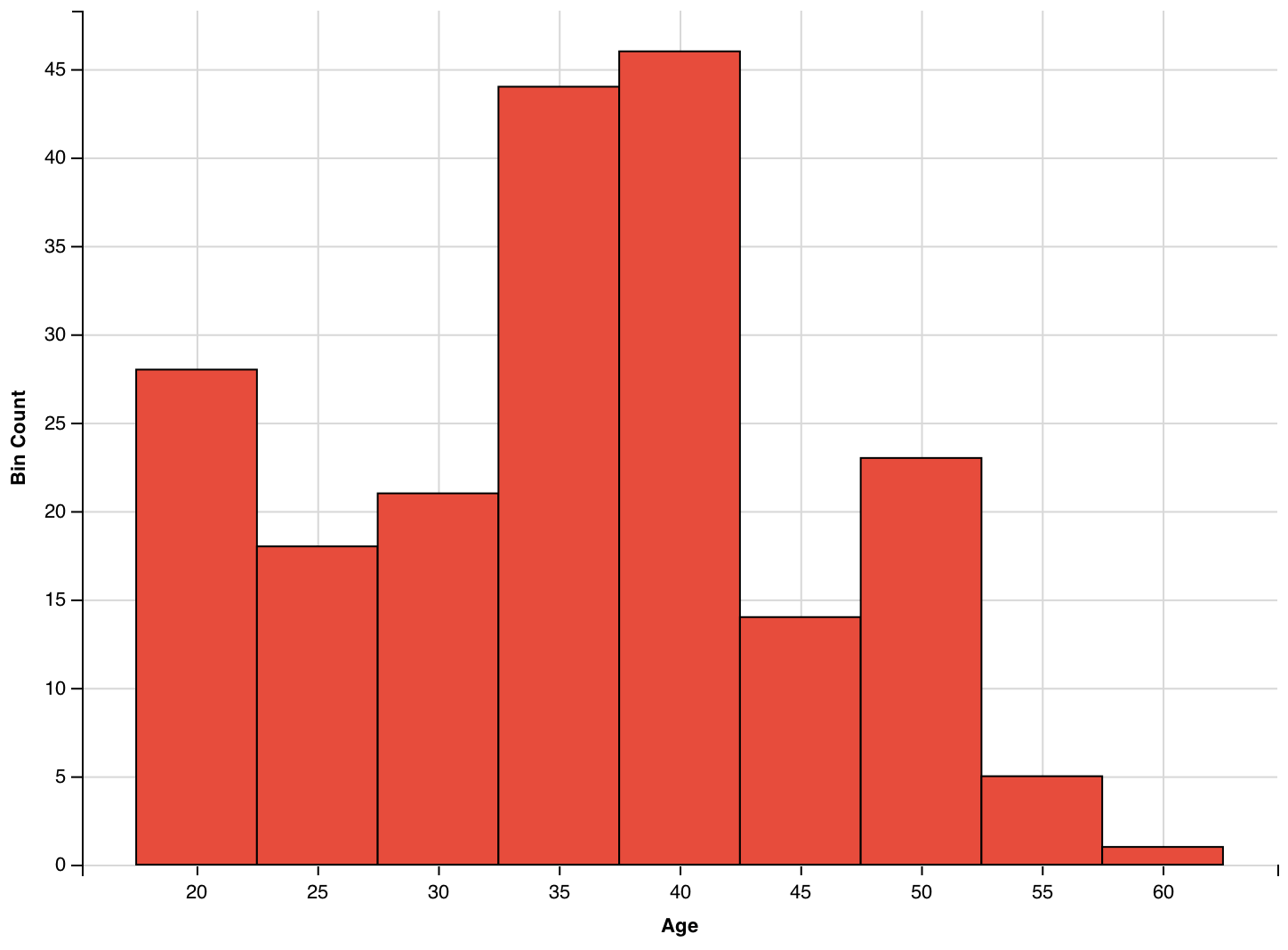Want to share your content on R-bloggers? click here if you have a blog, or here if you don't.

The two previous posts described how you can make histograms with basic R and the ggplot2 package. This third and last part of our histograms tutorial will look at how to make a histogram with ggvis. This package is similar to `ggplot2`, as it is also based on “the grammar of graphics”. However, `ggvis` has slightly different expressions and extends `ggplot2` by adding new features to make your plots interactive. Want to learn more? Discover the DataCamp tutorials.

## Step One. Get The ggvis Package into RStudio

To start off plotting histograms in `ggvis`, you first need to load the `ggvis` package:

```library(ggvis)
```

If `ggvis` is not yet installed on your system, you’ll have to do this first. This can easily be done through

```install.packages("ggvis")
```

It might seem silly, but do not forget about your data! You can use a data set that is built into R already, or get your own data set. In this example, we will continue with the data from the previous blogpost on histograms: the `chol` data set.

If you’re new to this tutorial, you can load in the data set through the `url()` function, embedded into the `read.table()` function:

```chol <- read.table(url("http://s3.amazonaws.com/assets.datacamp.com/blog_assets/chol.txt"), header = TRUE)
```

## Step Three. How to make a histogram with ggvis.

To make a basic histogram, you can use the following line of code:

```#Visualize the "AGE" column from the "chol" data frame in a simple histogram
chol %>%
ggvis(~AGE) %>%
layer_histograms()
```Study the line of code printed above a bit more clearly: you see that ggvis uses the operator `%>%`, which is also known as the pipe operator, from the `magrittr` package. This operator passes the result from its left-hand side into the first argument of the function on its right-hand side. So `f(x) %>% g(y)` is actually a shortcut for `g(f(x), y)`. As an extra example, consider the two following R commands, that are completely equivalent:

```option1 <- sum(abs(-3:3))
option2 <- -3:3 %>% abs() %>% sum()
```

## Step Four. Prettify Your Histograms

Similarly to the `hist()` function and functions of `ggplot2()`, you can easily adapt the visualization of your histogram by extending the original code. In this case, the original line of code could be extended as follows:

```#Visualize a histogram that takes the "AGE" column from the "chol" data set, fill the bins up with a red color and makes bins of width 5, with a zero center and add age as a title to the x-axis
chol %>%
ggvis(~AGE) %>%
layer_histograms(width = 5, center = 35, fill := "#E74C3C") %>%
```Just like with the `hist()` function and ggplot2, you can break up this rather large chunk of code to see what each small piece contributes to the visualization during the plotting of the histogram:

### Bins

You can easily adjust the width of the bins by changing the `width` argument inside the `layer_histograms()` function:

```chol %>%
ggvis(~AGE) %>%
layer_histograms(width = 5)
```The `width` argument already set the bin width to 5, but where do bins start and where do they end? You can use the `center` or `boundary` argument for this. `center` should refer to one of the bins’ center value, which automatically determines the other bins location. The `boundary` argument specifies the boundary value of one of the bins. Here again, specifying a single value fixes the location of all bins. As these two arguments specify the same thing in a different way, you should set at most one of `center` or `boundary`.

As an example, compare the previous histogram (with for example 35 as a bin center) to the following histogram, where `center` is set to 36:

```chol %>%
ggvis(~AGE) %>%
layer_histograms(width = 5, center = 36)
```You can enforce the same plot by setting the `boundary` argument (33.5 equals 36 minus half the bin width):

```chol %>%
ggvis(~AGE) %>%
layer_histograms(width = 5, boundary = 33.5)
```Note that the boundary and center may be outside the range of the data. In that case `ggvis` is smart enough to determine extrapolate what you meant and will decide on a location of the bins. Experiment with the arguments described above to see what the influence could be on the interpretation of the histogram.

### Names/colors

By using the pipe operator in addition to `add_axis()`, you can specify which axis you want to give a certain title or label. In this case, we put

```chol %>%
ggvis(~AGE) %>%
layer_histograms(width = 5, center = 35) %>%
```Similarly, you can also label the y-axis:

```chol %>%
ggvis(~AGE) %>%
layer_histograms(width = 5, center = 35) %>%
```You can fill up the bins with any color that you would like by using `fill :=`.

```chol %>%
ggvis(~AGE) %>%
layer_histograms(width = 5, center = 35, fill := "#E74C3C") %>%
```Note the use of `:=`, which sets a property to a specific color (or size, width, etc.), as opposed to *mapping* it, as the `=` operator does.

There are many advantages of working with ggvis, but one that definitely stands out is the fact that you can actually make your histograms interactive! This allows readers of your reports to change the parameters of your plots and see the results of these changes instantaneously. `ggvis` makes use of `shiny` for this. Let’s go into the basics of the interactivity that you can add to your histograms.

For example, you can add an input slider that lets you decide on the width of your bins. Here’s how:

```chol %>%
ggvis(~AGE) %>%
layer_histograms(width = input_slider(1, 10, step = 1, label = "Bin Width"),
center = 35,
fill := "#E74C3C") %>%
```Note that the figure that is shown in this post is a static version of the histogram. To check the interactive plot, that is backed with an R process to change the graphics on the fly, visit the histogram on our Shiny server.

The input values that are given to `input_slider` are those that you can also see when you execute the code in the RStudio console. In this case, you would see a slider that ranges from values 1 to 10 with steps of 1 and that has a label `Bin Width`.

Next, you can also add a different type of user input, a select box, to determine the fill color of the bins:

```chol %>%
ggvis(~AGE) %>%
layer_histograms(width = input_slider(1, 10, step = 1, label = "Bin Width"),
center = 35,
fill := input_select(choices = c("red", "green", "blue", "yellow"),
selected = "blue", label = "Fill Color")) %>%
```The interactive version of this histogram can again be found on our Shiny server. Here, the `fill` property is set to one of four choices, specified in the `choices` argument inside `input_select()`. `selected` specified that the default color is blue, while `label` shows the label `Fill Color` in the interactive plot.

Note that the shiny package is needed for these interactive visualizations. Normally, RStudio comes with this package by default. If you are not working in RStudio, install `shiny` by executing `install.packages("shiny")`.

Remember to keep in mind what you want to achieve with your histogram and how you want to achieve this! Sometimes, a static representation might be better than a dynamic one.

## Step Six. Using ggvis For So Much More

This section was just the tip of the iceberg! Believe us when we say that you can do so much more with this package.

Remember If you would like to have a more detailed and broader understanding of ggvis, you might be interested in the DataCamp R training path, which includes a ggvis course taught by Garrett Grolemund, author of Hands on Programming with R, as well as Data Science with R, an upcoming book from O’Reilly Media.

# Conclusion

There are a lot of options to make histograms in R. The option you should choose is really rather a trade-off between what you want to accomplish and how fast you want to accomplish this. But of course, this trade-off is subject to your own programming experience with R and other languages: either option would go fast for any well-trained programmer.

However, if you are just starting with R, it might be a good idea to keep this tutorial at hand. Additionally, we encourage you to go and check out the additional information that you can find in the tutorial and below. This way, you will gradually be submerged into the field of data visualization, which will give you the time to get fluent very quickly.

So, in the end, the only thing that remains of the original trade-off and that probably is the most important thing to take into account is the question “What do you want to achieve with your histogram?”.

The post How to Make a Histogram with ggvis in R appeared first on The DataCamp Blog .